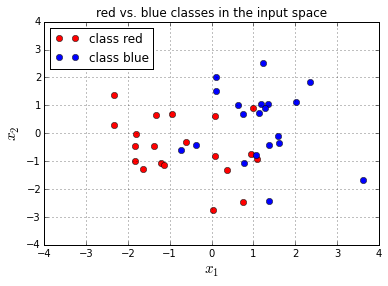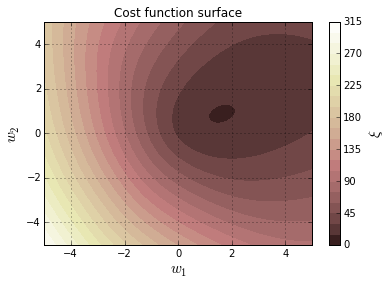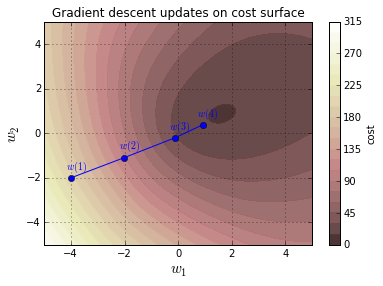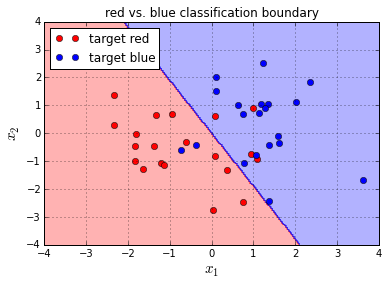Tags: , ,

# How to implement a neural network Intermezzo 1

http://peterroelants.github.io/posts/neural_network_implementation_intermezzo01/

This page is part of a 5 (+2) parts tutorial on how to implement a simple neural network model. You can find the links to the rest of the tutorial here:

## Logistic classification function

This intermezzo will cover:

If we want to do classification with neural networks we want to output a probability distribution over the classes from the output targets t

. For the classification of 2 classes t=1 or t=0

we can use the logistic function used in logistic regression . For multiclass classification there exists an extension of this logistic function called the softmax function which is used in multinomial logistic regression . The following section will explain the logistic function and how to optimize it, the next intermezzo will explain the softmax function and how to derive it.

In :

## Logistic function

The goal is to predict the target class t

from an input z. The probability P(t=1|z) that input z is classified as class t=1 is represented by the output y of the logistic function computed as y=σ(z). σ is the logistic function and is defined as:

σ(z)=11+ez

This logistic function, implemented below as logistic(z) , maps the input z

to an output between 0 and 1

as is illustrated in the figure below.

We can write the probabilities that the class is t=1

or t=0 given input z

as:

P(t=1|z)P(t=0|z)=σ(z)=11+ez=1σ(z)=ez1+ez

Note that input z

to the logistic function corresponds to the log odds ratio of P(t=1|z) over P(t=0|z)

.

logP(t=1|z)P(t=0|z)=log11+ezez1+ez=log1ez=log(1)log(ez)=z

This means that the logg odds ratio log(P(t=1|z)/P(t=0|z))

changes linearly with z. And if z=xw as in neural networks, this means that the logg odds ratio changes linearly with the parameters w and input samples x

.

In :

In :

### Derivative of the logistic function

Since neural networks typically use gradient based opimization techniques such as gradient descent it is important to define the derivative of the output y

of the logistic function with respect to its input z. y/z

can be calculated as:

yz=σ(z)z=11+ezz=1(1+ez)2ez1=11+ezez1+ez

And since 1σ(z))=11/(1+ez)=ez/(1+ez)

this can be rewritten as:

yz=11+ezez1+ez=σ(z)(1σ(z))=y(1y)

This derivative is implemented as logistic_derivative(z) and is plotted below.

In :

In :

### Cross-entropy cost function for the logistic function

The output of the model y=σ(z)

can be interpreted as a probability y that input z belongs to one class (t=1), or probability 1y that z belongs to the other class (t=0) in a two class classification problem. We note this down as: P(t=1|z)=σ(z)=y

.

The neural network model will be optimized by maximizing the likelihood that a given set of parameters θ

of the model can result in a prediction of the correct class of each input sample. The parameters θ transform each input sample i into an input to the logistic function zi

. The likelihood maximization can be written as:

argmaxθ(θ|t,z)=argmaxθi=1n(θ|ti,zi)

The likelihood (θ|t,z)

can be rewritten as the joint probability of generating t and z given the parameters θ: P(t,z|θ). Since P(A,B)=P(A|B)P(B)

this can be written as:

P(t,z|θ)=P(t|z,θ)P(z|θ)

Since we are not interested in the probability of z

we can reduce this to: (θ|t,z)=P(t|z,θ)=ni=1P(ti|zi,θ). Since ti is a Bernoulli variable , and the probability P(t|z)=y is fixed for a given θ

we can rewrite this as:

P(t|z)=i=1nP(ti=1|zi)ti(1P(ti=1|zi))1ti=i=1nytii(1yi)1ti

Since the logarithmic function is a monotone increasing function we can optimize the log-likelihood function argmaxθlog(θ|t,z)

. This maximum will be the same as the maximum from the regular likelihood function. The log-likelihood function can be written as:

log(θ|t,z)=logi=1nytii(1yi)1ti=i=1ntilog(yi)+(1ti)log(1yi)

Minimizing the negative of this function (minimizing the negative log likelihood) corresponds to maximizing the likelihood. This error function ξ(t,y)

is typically known as the cross-entropy error function (also known as log-loss):

ξ(t,y)=log(θ|t,z)=i=1n[tilog(yi)+(1ti)log(1yi)]=i=1n[tilog(σ(z)+(1ti)log(1σ(z))]

This function looks complicated but besides the previous derivation there are a couple of intuitions why this function is used as a cost function for logistic regression. First of all it can be rewritten as:

ξ(ti,yi)={log(yi)log(1yi)if ti=1if ti=0

Which in the case of ti=1

is 0 if yi=1 (log(1)=0) and goes to infinity as yi0 (limy0log(y)=+). The reverse effect is happening if ti=0.
So what we end up with is a cost function that is 0 if the probability to predict the correct class is 1 and goes to infinity as the probability to predict the correct class goes to 0

.

Notice that the cost function ξ(t,y)

is equal to the negative log probability that z is classified as its correct class:
log(P(t=1|z))=log(y),
log(P(t=0|z))=log(1y)

.

By minimizing the negative log probability, we will maximize the log probability. And since t

can only be 0 or 1, we can write ξ(t,y) as:

ξ(t,y)=tlog(y)(1t)log(1y)

Which will give ξ(t,y)=ni=1[tilog(yi)+(1ti)log(1yi)]

if we sum over all n

samples.

Another reason to use the cross-entropy function is that in simple logistic regression this results in a convex cost function, of which the global minimum will be easy to find. Note that this is not necessarily the case anymore in multilayer neural networks.

#### Derivative of the cross-entropy cost function for the logistic function

The derivative ξ/y

of the cost function with respect to its input can be calculated as:

ξy=(tlog(y)(1t)log(1y))y=(tlog(y))y+((1t)log(1y))y=ty+1t1y=yty(1y)

This derivative will give a nice formula if it is used to calculate the derivative of the cost function with respect to the inputs of the classifier ξ/z

since the derivative of the logistic function is y/z=y(1y)

:

ξz=yzξy=y(1y)yty(1y)=yt

This post at peterroelants.github.io is generated from an IPython notebook file. Link to the full IPython notebook file

# How to implement a neural network Part 2

http://peterroelants.github.io/posts/neural_network_implementation_part02/

This page is part of a 5 (+2) parts tutorial on how to implement a simple neural network model. You can find the links to the rest of the tutorial here:

## Logistic regression (classification)

This part will cover:

While the previous tutorial described a very simple one-input-one-output linear regression model, this tutorial will describe a 2-class classification neural network with two input dimensions. This model is known in statistics as the logistic regression model. This network can be represented graphically as:The notebook starts out with importing the libraries we need:

In :

## Define the class distributions

In this example the target classes t

will be generated from 2 class distributions: blue (t=1) and red (t=0). Samples from both classes are sampled from their respective distributions. These samples are plotted in the figure below. Note that X is a N×2 matrix of individual input samples xi, and that t is a corresponding N×1 vector of target values ti

.

In :

In :## Logistic function and cross-entropy cost function

### Logistic function

The goal is to predict the target class t

from the input values x. The network is defined as having an input x=[x1,x2] which gets transformed by the weights w=[w1,w2] to generate the probability that sample x belongs to class t=1. This probability P(t=1|x,w) is represented by the output y of the network computed as y=σ(xwT). σ is the logistic function and is defined as:

σ(z)=11+ez

This logistic function and its derivative are explained in detail in intermezzo 1 of this tutorial. The logistic function is implemented below by the logistic(z) method.

### Cross-entropy cost function

The cost function used to optimize the classification is the cross-entropy error function . And is defined for sample i

as:

ξ(ti,yi)=tilog(yi)(1ti)log(1yi)

Which will give ξ(t,y)=ni=1[tilog(yi)+(1ti)log(1yi)]

if we sum over all N

samples.

The explanation and derivative of this cost function are given in detail in intermezzo 1 of this tutorial. The cost function is implemented below by the cost(y, t) method, and its output with respect to the parameters w

over all samples x

is plotted in the figure below.

The neural network output is implemented by the nn(x, w) method, and the neural network prediction by the nn_predict(x,w) method.

In :

In :## Gradient descent optimization of the cost function

The gradient descent algorithm works by taking the derivative of the cost function ξ

with respect to the parameters, and updates the parameters in the direction of the negative gradient .

The parameters w

are updated by taking steps proportional to the negative of the gradient: w(k+1)=w(k)Δw(k+1). Δw is defined as: Δw=μξw with μ

the learning rate.

ξi/w

, for each sample i

is computed as follows:

ξiw=ziwyiziξiyi

Where yi=σ(zi)

is the output of the logistic neuron, and zi=xiwT

the input to the logistic neuron.

• can be calculated as:
ξiyi=yitiyi(1yi)
• can be calculated as:
yizi=yi(1yi)
• zi/w
• can be calculated as:
zw=(xw)w=x

Bringing this together we can write:

ξiw=ziwyiziξiyi=xyi(1yi)yitiyi(1yi)=x(yiti)

Notice how this gradient is the same (negating the constant factor) as the gradient of the squared error regression.

So the full update function Δwj

for each weight will become

Δwj=μξiwj=μxj(yiti)

In the batch processing, we just add up all the gradients for each sample:

Δwj=μi=1Nxij(yiti)

To start out the gradient descent algorithm, you typically start with picking the initial parameters at random and start updating these parameters according to the delta rule with Δw

until convergence.

is implemented by the gradient(w, x, t) function. Δw

is computed by the delta_w(w_k, x, t, learning_rate) .

In :

Gradient descent is run on the example inputs X

and targets t for 10 iterations. The first 3 iterations are shown in the figure below. The blue dots represent the weight parameter values w(k) at iteration k

.

In :

In :## Visualization of the trained classifier

The resulting decision boundary of running gradient descent on the example inputs X

and targets t

is shown in the figure below. The background color refers to the classification decision of the trained classifier. Note that since this decision plane is linear that not all examples can be classified correctly. Two blue dots will be misclassified as red, and four red spots will be misclassified as blue.

Note that the decision boundary goes through the point (0,0)

since we don’t have a bias parameter on the logistic output unit.

In :This post at peterroelants.github.io is generated from an IPython notebook file. Link to the full IPython notebook file

The next page is about How to implement a neural network Intermezzo 2

Pages: 1 2 3